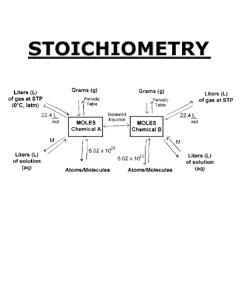# Aqueous Reactions And Solution Stoichiometry Test Prep.

25 Questions | Total Attempts: 490SettingsI made this to study from Mr. Guzman's Chem test! Whoop Whoop

• 1.
A solvent is present is _______ abundance
• A.

Average

• B.

Lowest

• C.

Greatest

• 2.
What are Electrolytes?
• A.

Substances that associate ions when poured/placed in water.

• B.

Substances that dissociate into ions when dissolved in water.

• C.

Substances that become strong acids, or strong bases when dissolved in water.

• 3.
Non-electrolytes ...
• A.

Remain unused at the end of the reaction

• B.

Are Weak Acids and Weak Bases

• C.

Are Usually a molecular compounds

• D.

Can dissolve in water, but no ions formed

• E.

Create a Salt

• F.

Causes the solution to indicate the presence of a base or acid.

• 4.
A Strong Electrolyte dissolves ____ when dissolved in water while a Weak electrolyte ___ dissolves.
• A.

Half, Completely

• B.

Partially,Completely

• C.

Completely, Partially

• D.

Partially, Half

• 5.
Which are the 7 Strong Acids?
• A.

HNa2

• B.

HCl

• C.

PClO2

• D.

HBr

• E.

HI

• F.

OH2

• G.

HNO4

• H.

HClO3

• I.

NaCl

• J.

HFO

• K.

HClO4

• L.

HNO3

• M.

Mg(OH)2

• N.

H2SO4

• 6.
Exceptions of soluble ionic compounds:NO3- : NoneC2H3O2- : Ag+
• A.

True

• B.

False

• 7.
Which three Soluble ionic compounds have the same exceptions?
• A.

NO3-, C2H3O2-, Ag+

• B.

Cl-, Br-, I-

• C.

SO4^2-, I-, S2-

• D.

OH, PO4^3-, CO3^2-

• 8.
What sign represents "soluble"
• A.

Aq

• B.

Liq.

• C.

D

• 9.
Which Ionic compound is soluble?Sodium Carbonate (Na2CO3)Lead (II) sulfatePbSO4
• A.

Sodium Carbonate (Na2CO3)

• B.

• C.

PbSO4

• 10.
What is formed when one mixes insoluble ions?
• A.

A double exchange reaction

• B.

A metathesis reaction

• C.

A precipitate

• D.

An acid

• 11.
What is another word for "Double Exchange Reaction"?
• A.

Precipitation Reaction

• B.

Single Replacement Reaction

• C.

Net Ionic Equation

• D.

Metathesis Reaction

• 12.
When does a Double Exchange Reaction usually occur?
• A.

When you react a Base with an Acid

• B.

When you mix two precipitates together

• C.

When you place electrolytes in a beaker of water

• 13.
What does an Ionic Equation do?
• A.

Takes out all of the insoluble compounds from the equation leaving you with the soluble ones.

• B.

Separates each soluble compound into its individual forms more accurately showing each ions species.

• C.

It cancels out the ions that are identical on both the reactant and product sides.

• 14.
A Net Ionic Equation crosses out what doesn't change from the left side to the right side of the equation.
• A.

True

• B.

False

• 15.
What is crossed out in a Net Ionic Equation
• A.

The Precipitate(s)

• B.

The Spectator Ion(s)

• C.

The Soluble Ion(s)

• 16.
Acids:
• A.

There are only 7 strong acids

• B.

Increase the concentration of H+ when dissolved in water

• C.

Decrease the concentration of H+ when dissolved in water

• D.

Are Proton-Acceptors

• E.

Are Proton-Donors

• F.

There are only 9 Strong Acids

• 17.
Bases:
• A.

All elements on column 1 along with Cl, Br, and I

• B.

Increase the concentration of OH- when dissolved in water

• C.

Decrease the concentration of H+ when dissolved in water

• D.

Are Proton-Acceptors

• E.

Are Proton-Donors

• F.

The strong bases are all alkali metals, Calcium, Strontium, and Barium

• 18.
What are the products of an Acid-Base reaction?
• A.

Spectator Ions

• B.

Salt and a Precipitate

• C.

Salt and Water

• 19.
A acid-base solution that produces a salt and water is also known as a ___________________
• 20.
When does an Oxidation-Reduction Reaction Occur?
• A.

Single replacement reaction

• B.

Double replacement reaction

• C.

When spectator ions are formed

• 21.
What 2 things react in a single replacement reaction?
• A.

A salt and water

• B.

A strong acid, and a strong base

• C.

An element, and an ion

• 22.
When do you use the activity Series?
• A.

To determine if the reaction can take place in a Double Replacement Reaction

• B.

To determine if the reaction can take place in a Single Replacement Reaction

• C.

To determine if the reaction can take place in an Acid Base Reaction

• 23.
What is Mr. Guzman's molarity Equation?
• A.

M = (Moma) x (L of Sol) / L of Solution = Mass Moles/ L solution of (g)

• B.

M = moles solute / L of solution = Mass (g) Solution / ((Moma of Solute)x(L of Sol))

• C.

M = L of Solution / Mass (g) of Solution = Moles Solute / (Moma of Solution)x(L of Sol)

• 24.
When is this equation used?Mi x Vi = Mf x Vf
• A.

To determine Dilution

• B.

To determine an Acid

• C.

To determine an Indicator

• 25.
When taking the test, if you run into a Dilution problem, the variables are most likely not going to be in order of Mi x Vi = Mf x Vf
• A.

True

• B.

False

Related TopicsBack to top